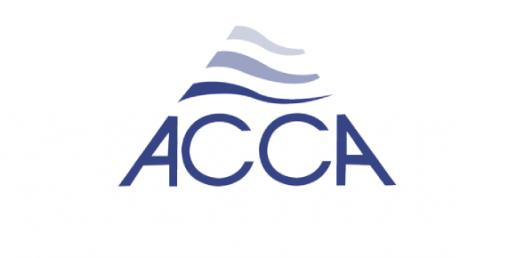# ACCA F2: Accounting For Materials Trivia Questions! Quiz

10 Questions | Attempts: 2215SettingsEnter your name and email address to take the Free MCQ Test on Accounting for Materials (ACCA F2 / FIA FMA). Number of Questions: 10 questions Time Available: 24 minutes Your score will be available instantly on an online certificate followed by the correct answers to all questions.

• 1.
The purchase price of an inventory item is \$25 per unit. In each three month period the usage of the item is 20,000 units. The annual holding costs associated with one unit equate to 6% of its purchase price. The cost of placing an order for the item is \$20. What is the economic order quanity for the inventory item to the nearest whole unit?
• A.

730

• B.

894

• C.

1,461

• D.

1,633

• 2.
A company always determines its order quantity for a raw material by using the EOQ model. What would be the effects on the EOQ and the total annual holding cost of a decrease in the cost of ordering a batch of raw material?
• A.

EOQ: Higher, Annual Holding Cost: Lower

• B.

EOQ: Higher, Annual Holding Cost: Higher

• C.

EOQ: Lower, Annual Holding Cost: Higher

• D.

EOQ: Lower, Annual Holding Cost: Lower

• 3.
Sky Limited wishes to minimise its inventory costs. At the moment its reorder quantity is 1,000 units. Order costs are \$10 per order and holding costs are \$0.10 per unit per month. Sky limited estimates annual demand to be 15,000 units. What is the optimal reorder quantity (to the nearest 100 units)?
• A.

500 units

• B.

1,000 units

• C.

1,200 units

• D.

1,700 units

• 4.
A company uses 9,000 units per annum. The component has a purchase price of \$40 per unit and the cost of placing an order is \$160. The annual holding cost of one component is equal to 8% of its purchase price. What is the EOQ (to the nearest unit) of the component?
• A.

530

• B.

671

• C.

949

• D.

1,342

• 5.
A company determines its order for a component using EOQ. What would be the effects on the EOQ and the total annual ordering cost of an increase in the annual cost of holding one unit of the component in inventory?
• A.

EOQ: Lower, Total Annual Ordering Cost: Higher

• B.

EOQ: Higher, Total Annual Ordering Cost: Lower

• C.

EOQ: Lower, Total Annual Ordering Cost: No Effect

• D.

EOQ: Higher, Total Annual Ordering Cost: No Effect

• 6.
The demand for a product is 12,500 units for a three month period. Each unit of product has a purchase price of \$15 and ordering costs are \$20 per order placed. The annual holding cost of one unit of product is 10% of its purchase price. What is the EOQ (to the nearest unit)?
• A.

577

• B.

1,816

• C.

1,866

• D.

1,155

• 7.
The demand for an item of material is 8,000 units each year. The cost of making an order is \$240. The purchase cost per unit is \$9 and the holding cost of inventory is 6% per annum of the purchase cost. Using the economic order quantity formula, what quantity of the item should be purchased in each order (to the nearest unit)?
• A.

800

• B.

843

• C.

1,886

• D.

2,667

• 8.
A company uses the EOQ formula to decide the purchase quantity for materials. The purchase cost of item 1234 is \$25 per unit. Annual demand is 10,000 units. The annual holding cost is \$2.50 per unit and ordering costs are \$500 per order. The supplier is offering a reduction in the purchase price to \$23 per unit on all orders of 5,000 units or more. Which one of the following statements is correct?
• A.

The company will minimise its total annual costs if it continues to purchase in the EOQ of 1,000 units per order.

• B.

The company will minimise its total annual costs if it continues to purchase in the EOQ of 2,000 units per order.

• C.

The company will minimise its total annual costs if it takes the larger order discount and purchases in quantities of 5,000 units per order.

• D.

The company will minimise its total annual costs if it takes the larger order discount and purchases in quantities of 10,000 units per order.

• 9.
A company manufactures Product Z in batches. Output capavity is 1,000 units of Product Z per week but demand is only 200 units per week. There are 50 weeks in each year. The annual cost of holding finished units of Product Z in store is \$2 per unit per year. Set-up costs for a batch of Product Z are \$800 per batch. What batch production quantity for Product Z will minimise total annual costs, to the nearest 100 units?
• A.

400 units

• B.

2,800 units

• C.

3,200 units

• D.

7,100 units

• 10.
A production department uses Component P to manufacture a product. This component is purchased from an external supplier in quantities of 3,000 units per order. Daily usage is 150 units of the component each day. The supply lead time is normally 6 days, but might be as little as 4 days or as much as 10 days. To avoid the risk of running out of inventory of Component P, what should be the reorder level for this item?
• A.

3,900 units

• B.

1,500 units

• C.

900 units

• D.

600 units

## Related TopicsBack to top
×

Wait!
Here's an interesting quiz for you.